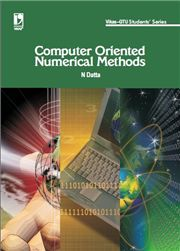# Computer Oriented Numercial Methods (GTU), 1/e N Datta-S.CHAND

250.00 225.00

Computer Arithmetic
• Iterative Methods
• Interpolation and Approximation
• Numerical Differentiation and Integration
• Matrix
• Numerical Solution of a System of Linear Equations
• Numerical Solution of Ordinary Differential Equation